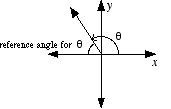index: click on a letter A B C D E F G H I J K L M N O P Q R S T U V W X Y Z A to Z index index: subject areas numbers & symbols sets, logic, proofs geometry algebra trigonometry advanced algebra & pre-calculus calculus advanced topics probability & statistics real world applications multimedia entrieswww.mathwords.com about mathwords website feedback

 Reference Angle For any given angle, its reference angle is an acute version of that angle. In standard position, the reference angle is the smallest angle between the terminal side and the x-axis. The values of the trig functions of angle θ are the same as the trig values of the reference angle for θ, give or take a minus sign.